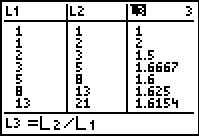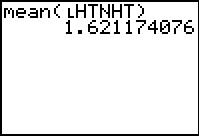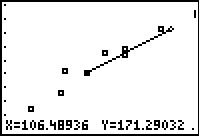# Activities

••• ##### Subject Area

• Math: Middle Grades Math: Algebraic Thinking

• ##### Author6-8

45 Minutes

• ##### Device
• TI-73 Explorer™
• TI-Navigator™

## Golden Ratio#### Activity Overview

Students will investigate Fibonacci numbers and the ratios of successive Fibonacci numbers. They will take measurements of their height and their navel heights and investigate the relationship between the two measurements.

#### Key Steps

•In this activity, students will investigate Fibonacci numbers and the ratios of successive Fibonacci numbers. They will take measurements of their height and their navel heights and investigate the relationship between the two. Finally, they will examine a scatter plot and a linear relationship fitted to the data points.

•First, students enter the first 20 Fibonacci numbers in L1 and the 2nd through 21st numbers in
L2 to calculate the ratio in L3.

Then, students gather height versus navel height data and calculate the mean of the class data. They compare this to the Golden ratio.

•Finally, students plot the data and use the Manual-Fit command to draw a line of best fit.